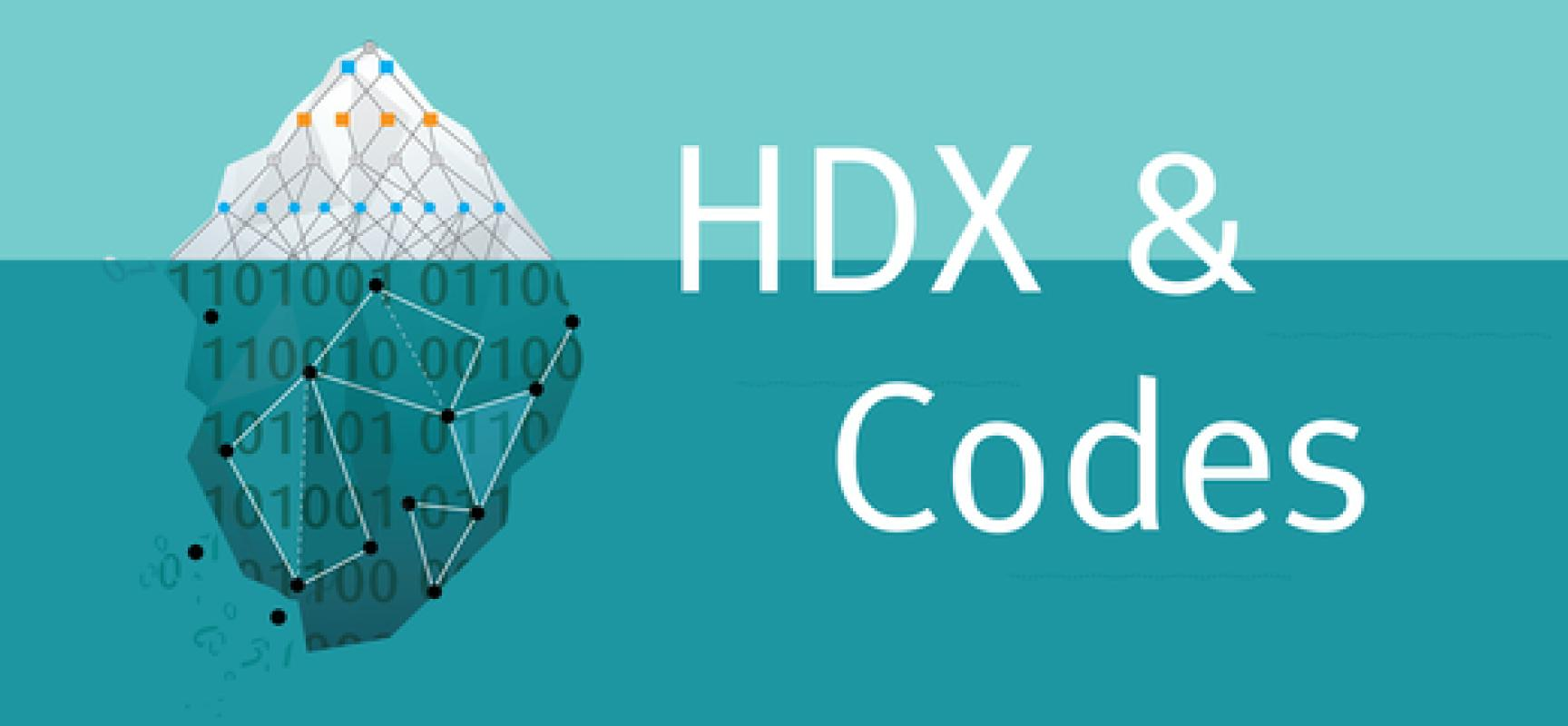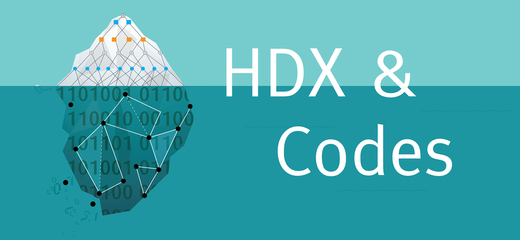# Research Vignette: The Many Dimensions of High-Dimensional ExpandersAn emerging theme in several disciplines of science over the last few decades is the study of local-to-global phenomena. Let me elucidate with a few examples. In biology, one tries to understand the global properties of an organism by studying local interactions at a cellular level. The Internet graph is impossible to predict or control at a global level; what one can at best do is understand its behavior by making changes at a very local level. Moving to examples closer to theoretical computer science, the pioneering works in computation theory due to Turing and others define the computation of any global function as one that can be broken down into a sequence of local computations. The seminal work on NP-completeness due to Cook, Levin, and Karp demonstrates that any verification task can be in fact reduced to a conjunction of verifying very local objects.

Given these examples, an intriguing question that arises in multiple disciplines is to identify which objects support such local-to-global phenomena. This question as stated is an ill-formed one, but one can attempt to make it well-defined in a variety of contexts. Let me illustrate by giving three specific instances.

Mixing time in graphs: Which graphs have the property of having a global mixing property that can be inferred by understanding the mixing properties of several related smaller graphs?

Local testing and decoding (in coding theory): Which error-correcting codes have the property of being able to be tested or decoded by looking at the code word or received word very locally (i.e., at very few locations)?

Topologically expanding graphs: Which graphs or hypergraphs have the property that their global topological property can be inferred by their local topological behavior?

These questions, seemingly disparate and arising in very different contexts and communities (in particular, approximate sampling, coding theory, and topology), surprisingly all led to the investigation of a similar expanding object: the high-dimensional expander. The Simons Institute 2019 summer cluster on Error-Correcting Codes and High-Dimensional Expansion brought together researchers from these diverse communities to study high-dimensional expanders and their potential applications to mathematics and theoretical computer science.

What is a high-dimensional expander?

High-dimensional expanders (HDXs) are a high-dimensional analogue of expander graphs. An expander graph, loosely speaking, is an extremely well-connected graph. Analytically, this is best captured via the second-largest eigenvalue (in absolute value) of the normalized adjacency matrix of the graph. More precisely, a graph G is said to be a λ-expander (for λ ∈ [0,1]) if the second-largest eigenvalue (in absolute value) of the normalized adjacency matrix AG of G is bounded above by λ. The smaller the λ, the better the expander. An equivalent definition is in terms of how well the random walk on the vertices induced by the graph mixes: G is a λ-expander if the spectral norm of the difference of the normalized adjacency matrix AG and the normalized all-ones matrix J (the normalized adjacency matrix of the complete graph) is bounded above by λ (i.e., $\left\| A_G - \frac1n J \right\| \leq \lambda,$

where n is the number of vertices of the graph G).

High-dimensional expanders refer to a particular generalization of expander graphs to higher dimensions (or hypergraphs). Given a d-uniform hypergraph H = (V,E) where $$E \subseteq {V \choose d}$$, for 0 ≤ dk let Hk denote the k-uniform hypergraph (V,Ek) whose hyperedges Ek are exactly the k-sets contained in some hyperedge e in E. In other words, $E_k = \left\{ e \in {V \choose k} \mid \exists e' \in E, e \subseteq e' \right \}.$

There are two natural random walks on the hyperedges Ek of the hypergraph Hk for each 0 < k < d.

Up-down walk $$P_k^\wedge$$: Given a hyperedge eEk, choose a random e′ ∈ Ek+1 that contains e, and then choose a random $$f \in E_k$$ such that f is contained in e′ and fe.

Down-up walk $$P_k^\vee$$: Given a hyperedge eEk, choose a random e′ ∈ Ek−1 contained in e, and then choose a random  $$f \in E_k$$ such that f contains e′ and fe.

The hypergraph H is said to be a λ-high dimensional expander if for all 0 < k < d, the two walks $$P_k^\wedge$$ and $$P_k^\vee$$ (viewed as transition probability matrices on the hyperedges) are λ-close to each other in spectral norm, i.e., $\left\| P_k^\wedge - P_k^\vee \right\| \leq \lambda.$

A local-to-global connection discovered a couple of years ago (independently by Kaufman and Oppenheim and in joint work by the author with Dikstein, Dinur, and Filmus) showed that this “global” mixing of the hypergraph at every level is in fact equivalent to the “local” mixing of several related smaller underlying graphs of the hypergraph H (also referred to as links of the hypergraph H).

Which hypergraphs are high-dimensional expanders in the above sense? Clearly, the complete k-uniform hypergraph is a perfect high-dimensional expander. Unlike in the case of graphs, where expansion is a common phenomenon, for all d ≥ 3, a random d-uniform hypergraph is with high probability not a high-dimensional expander. Despite this, a remarkable construction due to Lubotzky, Samuels, and Vishne building on the work of Lafforgue shows that there exist extremely sparse hypergraphs (in fact, with at most a linear number of hyperedges) that satisfy the above high-dimensional property. Since then, elementary constructions of sparse high-dimensional expanders have been constructed by Kaufman and Oppenheim, whose proofs were subsequently simplified during the Simons Institute summer cluster, in joint work with Saptharishi.

In fact, with the benefit of hindsight, if one revisits the locally testable code (LTC) and probabilistically checkable proof (PCP) constructions to date, one notices that a common underlying object in all these constructions is certain high-dimensional expanding objects. In particular, all known constructions of these objects are based on either the Johnson graph or the Grassmannian graph, and it is known that these two graphs are classic examples of high-dimensional expanders. We believe that this is not a coincidence and that high-dimensional expanders play a fundamental role in PCP and LTC constructions.

One of the prime motivations for the Simons Institute cluster was to explore these connections further and to investigate whether the recent abovementioned sparse constructions of high-dimensional expanders have consequences in coding theory. Recent works coauthored by Dinur, Harsha, Kaufman, Navon, and Ta-Shma, and by Alev, Jeronimo, Quintana, Srivastava, and Tulsiani, demonstrate the effectiveness of sparse HDXs toward obtaining efficient list-decoding algorithms. In work by Dikstein, Dinur, Harsha, Kaufman, and Ron-Zewi during the Simons cluster, HDXs were used to obtain better testable codes. The connections between HDXs and coding theory are at a nascent stage, and we believe the results obtained to this day are only the tip of the iceberg.

Anari, Liu, Oveis Gharan, and Vinzant discovered a serendipitous connection between approximate sampling of the bases of a matroid and high-dimensional expanders. Approximately counting or sampling the bases of a matroid has been a long-standing open problem in theoretical computer science. Anari et al. made the surprising connection that the hypergraph whose edges are the independent sets of a matroid is an excellent high-dimensional expander. Thus, the down-up walk described earlier gives a very natural fast-mixing sampling algorithm. This is an excellent example of a case where one understands the global spectrum/mixing of a large graph by relating it to the mixing/spectrum of several related small local graphs. Since the discovery of this connection, Cryan, Guo, and Mousa, as well as Alev and Lau, have made substantial refinements to this local-to-global phenomenon in understanding the mixing of these random walks.

This understanding of local-to-global phenomenon in nature is a fundamental problem, and the theory of high-dimensional expanders offers an excellent perspective on this behavior in some instances.

,